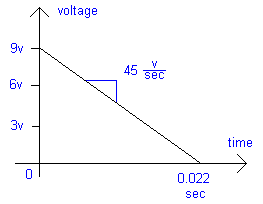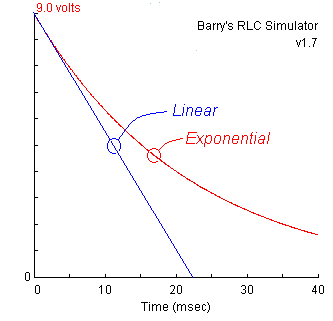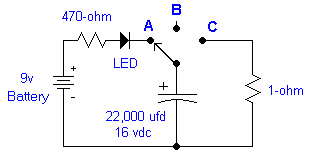# Capacitor Charge and Discharge

What happens when a capacitor is charging? How does charging really work? How does it discharge? Let's take a close look at the basics. To help concentrate on the capacitor we assume the load is purely resistive, and ignore any effects of an attached inductor.

 Key Principle: The guiding rule of nature is that current is the same everywhere in a series circuit. Charges will move around, but are neither created nor destroyed. For charges to move, they must move identically everywhere in a series circuit.

##Discharge

Example: Suppose your capacitor is charged to 9 volts, and at time t = 0 the switch is connected to a one ohm resistor. The discharge time is regulated by the resistance.

The initial current (t = 0) is I = V/R = (9 volts)/(1 ohm) = 9 amps.For a moment, let's assume the rate of discharge is constant. That is, it will follow a linear discharge curve over time. At this rate it would discharge in time:
t = C * V / I = (0.022)*(9 volts)/(9 amps) = 0.022 sec = 22 milliseconds.

But! The rate is not actually linear. Our assumption was wrong, because the current drops as the voltage drains away. This means it discharges at a progressively slower rate over time. When the capacitor voltage reaches 6 volts, there will only be 6 amps. When it's 3 volts, the current is 3 amps. When it's down to 1 mV there's only 1 mA! An ideal capacitor will never completely discharge! It will gradually approach zero volts but never quite reach it.

## Exponential Decay

Exactly how does a capacitor discharge? From above, we see that capacitors do not discharge at a linear rate through resistors.

When you do the math for a capacitor discharge, you get an exponential decay curve: V(t) = V0 e-t / RCThis curve starts at the initial capacitor voltage (V0), and diminishes quickly at first. As time goes on, the slope becomes less and less while the voltage approaches (but does not reach!) zero. However, for all practical purposes the capacitor might as well be empty by the time 99% of the initial charge has escaped.

Shown at left is a comparison between a linear and exponential decay for this circuit. The exponential curve is a screen shot taken from the RLC Simulator applet.

This graph shows that an exponential decay curve at 22 msec is only 64% discharged. Not only is it non-zero, but it isn't even 2/3 discharged yet! For this circuit at 40 msec, the exponential decay curve still has 16% of the original charge remaining.

Does a charged capacitor complete a circuit, or does it slowly discharge? In the figure at right, a switch connects a capacitor to (a) a battery, then (b) unconnected, and finally (c) a low resistance. Let's study what happens in each position.

## (A) ChargeWith the switch at A, the capacitor is charging. Current flows from the battery through the capacitor. The electrons move to one plate, but they do not jump the insulating gap inside the capacitor. They collect on the surface of the plate.

Meanwhile, electrons are removed from the other plate from the abundance that is always there in metals. That gives the plate a net positive charge. And removing the charge completes the path around which current flows.

The current is always the same on both terminals of a capacitor. You can't move charge into one terminal without removing it from the other.

As the current flows from the battery to the capacitor, it travels through the LED. This emits light during the charging cycle, and then dims and finally turns dark when the capacitor is fully charged.

## (B) DisconnectedWith the switch at B, the capacitor is disconnected. What happens? There is no current on one terminal of the capacitor. There there must be no current on the other terminal.

With no current flowing, the capacitor will keep its 9-volt charge nearly forever. It is stored in the electric field between the two places. It cannot move due to the insulator -- the charges cannot jump the gap.

In practice, no insulator is perfect and the charge will eventually leak away. But this may take months in a high-quality capacitor. Additionally, a significant charge can remain forever, stored in the chemical reaction that ionizes the plate surfaces of an electrolytic capacitor. Be wary of old capacitors because they can bite!

## (C) DischargeWith the switch at C, the capacitor is connected to the 1-ohm resistor. What happens? The charges stored in the capacitor's electric field now have an escape route. They can finally flow from one plate to the other, by travelling through the resistor.

The rate of charge travel (current) depends on the circuit resistance, and alos on how hard it is pushed by the strength of the internal electric field (voltage).

Q: Does the capacitor need to discharge back to the battery, or to itself?
A: Neither. It can only discharge by being connected to something with a lower voltage, such as a resistor or coil. When you move the switch to A then current will flow from the battery to the capacitor until their voltages are equal again.

Q: Where does it go? Can we run the current back into the capacitor, essentially recharging itself, or must it go back into the battery?
A: The current (and capacitor's voltage) is gone. It was transformed into a small amount of heat in the resistor.

Q: How does the battery handle the power spike when the capacitor is connected?
A: Easily. When a battery (or capacitor) is connected to something with a lower voltage, current will flow. Batteries generate current from an internal chemical reaction. Eventually all the chemicals finish combining with each other, and you need to recharge (or recycle) the battery.

## Current Myth

Electrons doesn't flow as fast or far as you might expect! Metal conductors contain a vast ocean of electrons, and only a small number drift a short distance to provide all the current you need. This is a common misconception that is glossed over in practically all text books (and my web pages too!).
See "What Is Electricity?" by Bill Beatty to debunk some common misconceptions.

 < Previous Page 4 of 6 Next >